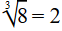# Surds

Contents

## Summary

• Rational Number: A number that can be written as a fraction, where both the numerator and the denominator are whole numbers. E.g 3/4
• Irrational Number: They cannot be written as a fraction. They can only be written as decimals numbers. E.g$\sqrt { 3 }$  which is equal to 1.7320……
• A surd is a number that’s written with the$\sqrt$  (under root) sign. Remember the answer has to be irrational.
• Rules for using surds:
i)$\sqrt { ab } \quad =\quad \sqrt { a } \quad\sqrt { b }$
ii)$\sqrt { \frac { a }{ b } } \quad =\quad \frac { \sqrt { a } }{ \sqrt { b } }$
iii)$a\quad =\quad { (\sqrt { a } ) }^{ 2 }\quad =\quad \sqrt { a } \quad \sqrt { a }$

Before we move on to surds, let us recall what rational and irrational numbers are.

#### Rational Number

A rational number is a number that can be written as a fraction, where both the numerator and the denominator are whole numbers.

Every whole number is a rational number, because any whole number can be written as a fraction i.e number 8 is a rational number because it can be written as the fraction 8/1.

Some other examples of rational numbers include:

• 3/4
• 25, 144, 808/56, 103, 492
• 1/2 etc

#### Irrational Numbers

Numbers that are not rational are considered irrational. Irrational numbers cannot be written as a fraction. They can only be written as decimals numbers.

Moreover, they have endless digits to the right of the decimal point.

Some examples of irrational numbers include:

• The value of$\pi$  which is equal to 3.1415…….
•$\sqrt { 3 }$  which is equal to 1.7320……$\sqrt { 4 }$   is not a irrational number as it is equal to 2 ( a whole number).

Now that we have revised what rational and irrational numbers are, let’s move on to Surds.

#### What are surds?

A surd is a number that’s written with the$\sqrt$  (under root) sign. It can be a square root,  cube rootor other n roots.

These are used when an exact answer is required i.e if the answer is$\sqrt { 3 }$,  we can leave it like this instead of solving this on a calculator to give an answer in decimals after rounding off like 1.732.

No matter how many decimal places we use, we can never get exactly 3 after squaring 1.7320….. We will end up getting something like 2.99999 which is not very accurate.

Hence, we say that Irrational root of a rational positive number is called a surd. I.eor$\sqrt { 2 }$ . Remember the answer has to be irrational, for instance$\sqrt { 4 }$  andare not surds as they result in a rational number:$\sqrt { 4 } \quad =\quad 2$  and.

#### Rules for using Surds$\sqrt { ab } \quad =\quad \sqrt { a } \quad\sqrt { b }$$\sqrt { \frac { a }{ b } } \quad =\quad \frac { \sqrt { a } }{ \sqrt { b } }$$a\quad =\quad { (\sqrt { a } ) }^{ 2 }\quad =\quad \sqrt { a } \quad \sqrt { a }$

#### Example #1

Q. Simplify$\sqrt { 12 }$

Solution:$\sqrt { 12 } \quad =\quad \sqrt { 4\quad \times \quad 3 } \quad =\quad 2\sqrt { 3 }$

Q. Find${ (2\sqrt { 5 } \quad +\quad 3\sqrt { 6 } ) }^{ 2 }$

Solution:${ (2\sqrt { 5 } \quad +\quad 3\sqrt { 6 } ) }^{ 2 }\quad =\quad (2\sqrt { 5 } \quad +\quad 3\sqrt { 6 } )(2\sqrt { 5 } \quad +\quad 3\sqrt { 6 } )$$=\quad { (2\sqrt { 5 } ) }^{ 2 }\quad +\quad 2\quad \times \quad 2\sqrt { 5 } \quad \times \quad 3\sqrt { 6 } \quad +\quad { (3\sqrt { 6 } ) }^{ 2 }$$=\quad ({ 2 }^{ 2 }\quad\sqrt { { 5 }^{ 2 } } )\quad +\quad 2\times2\quad \times 3\times \quad \sqrt { 5 } \quad \times \quad \sqrt { 6 } \quad +\quad ({ 3 }^{ 2 } \quad \sqrt { { 6 }^{ 2 } } )$$=\quad 20\quad +\quad 12\sqrt { 30 } \quad +\quad 54$$=\quad 74\quad +\quad 12\sqrt { 30 }$

Q. Rationalise the denominator of$\frac { 1 }{ 1\quad +\quad \sqrt { 2 } \quad }$

Solution:

Firstly multiply the top and bottom by the denominator, but change the sign in front of the surd.$\quad =\quad \frac { 1 }{ 1\quad +\quad \sqrt { 2 } \quad } \quad \times \quad \frac { 1\quad -\quad \sqrt { 2 } }{ 1\quad -\quad \sqrt { 2 } }$$=\quad \frac { 1\quad -\quad \sqrt { 2 } }{ (1\quad +\quad \sqrt { 2 } )(1\quad -\quad \sqrt { 2 } )\quad }$$=\quad \frac { 1\quad -\quad \sqrt { 2 } }{ { 1 }^{ 2 }\quad +\quad \sqrt { 2 } \quad -\quad \sqrt { 2 } \quad -\quad \sqrt { { 2 }^{ 2 } } \quad }$$=\quad \frac { 1\quad -\quad \sqrt { 2 } }{ 1\quad -\quad 2 } \quad =\quad \frac { 1\quad -\quad \sqrt { 2 } }{ -1 }$

Ans:$=\quad -1\quad +\quad \sqrt { 2 }$

##### Reference
1. https://www.factmonster.com/math/numbers/rational-and-irrational-numbers
2. CGP Edexcel AS-Level Mathematics , Complete Revision and Practice.